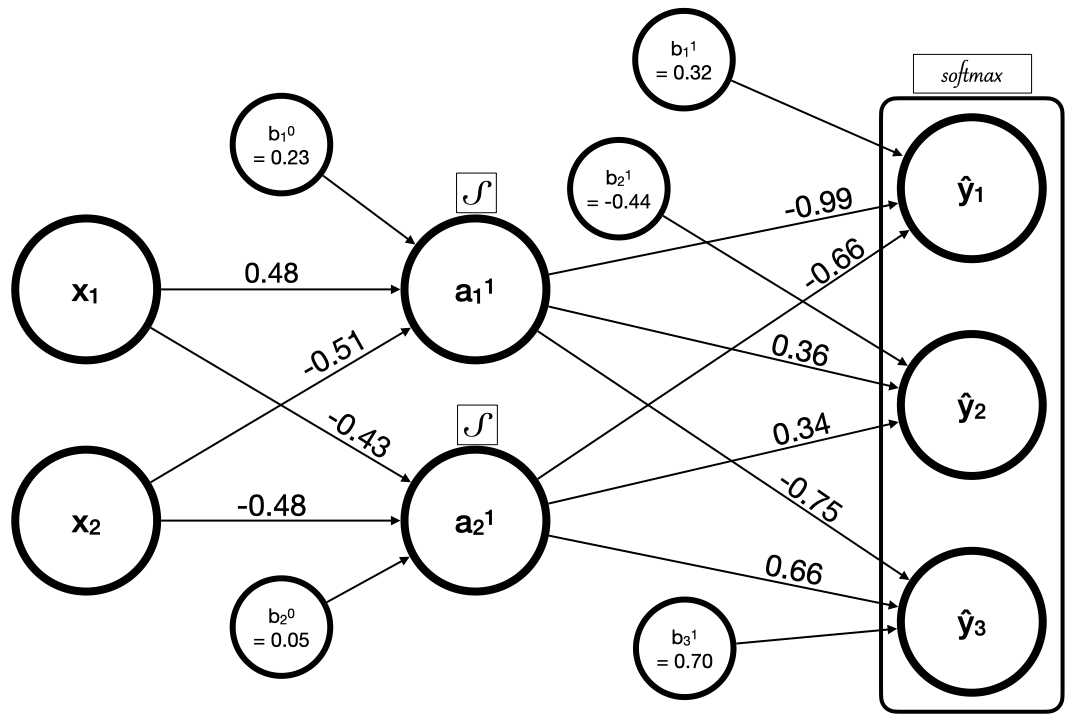# Vanilla Neural Network Problem¶

Here's a "vanilla"feed-forward neural network with logistic activation functions and softmax applied to the output layer.Given the following X and y tensors representing training data,

import torch

# Make data
torch.manual_seed(4321)
X = torch.rand(size=(8,2))
y = torch.randint(low=0, high=3, size=(8,))

print(X)
# tensor([[0.1255, 0.5377],
#         [0.6564, 0.0365],
#         [0.5837, 0.7018],
#         [0.3068, 0.9500],
#         [0.4321, 0.2946],
#         [0.6015, 0.1762],
#         [0.9945, 0.3177],
#         [0.9886, 0.3911]])

print(y) # (1)!
# tensor([0, 2, 2, 0, 2, 2, 0, 1])

1. y identifies the correct class label from a set of three possible labels: {0, 1, 2}.

calculate:

1. the predictions (forward pass)
2. the loss using categorical cross entropy
3. the gradient of the loss with respect to the weights and biases

### Formulas¶

Logist Function

$f(x)={\frac {1}{1+e^{-x}}}$

Softmax Function

$\sigma (\mathbf {z} )_{i}={\frac {e^{z_{i}}}{\sum _{j=1}^{K}e^{z_{j}}}}\ \ \ \ {\text{ for }}i=1,\dotsc ,K{\text{ and }}\mathbf {z} =(z_{1},\dotsc ,z_{K})\in \mathbb {R} ^{K}.$

Categorical Cross Entropy Loss

{\begin{aligned}J(\mathbf {w} )\ =\ -{\frac {1}{N}}\sum _{n=1}^{N}\ {\bigg [}y_{n}\log {\hat {y}}_{n}+(1-y_{n})\log(1-{\hat {y}}_{n}){\bigg ]}\,,\end{aligned}}